# GED Math : Circles

## Example Questions

← Previous 1 3 4 5 6 7 8 9 17 18

### Example Question #1 : Radius And Diameter

What is the radius of a circle with a diameter of 12 inches?Explanation:

The radius is half of the diameter: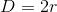From here we plug in our diameter measure of 12 and solve for the radius: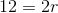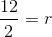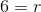### Example Question #2 : Circles

What is the diameter of a circle with radius of 10 inches?Explanation:

The diameter is twice the radius: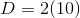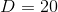### Example Question #1 : 2 Dimensional Geometry

What is the radius of a circle that has a circumference of?

18

20

12

4

18

Explanation:

The equation for circumference of a circle is as follows:Plug in the given values and solve for the radius: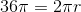Now we divide each side by: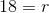### Example Question #1 : Circles

Find the diameter of a circle that has a circumference of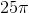?

20

12.5

10

25

25

Explanation:

We know the equation for the circumference of a circle is. We also know that the the diameter is the twice the radius, so we can write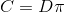.

Plug in the given values and solve: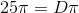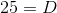### Example Question #1 : Radius And Diameter

What is the diameter of a circle with a radius of 9?

16

20

18

6

18

Explanation:

The diameter of a circle is twice the radius: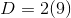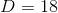### Example Question #1 : Radius And Diameter

What is the radius of a circle given the diameter is 22?

11

9

6

15

11

Explanation:

The radius is half of the diameter, or 11.

### Example Question #7 : Circles

What is the diameter of a circle with an area of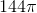?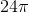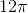Explanation:

In order to solve this question, start by finding the radius.  Recall that the area of a circle is computed as:For our data, this is: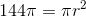Solving for, we get: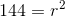or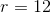Now, recall that the diameter is double the radius.  Thus, it is.

### Example Question #8 : Circles

What is the radius of a circle with a circumference of?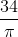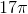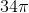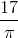Cannot be computed from the information providedExplanation:

Recall that the circumference formula is:Now, for your data, this gives you: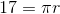You need to be careful, for some students get confused when they have to divide by.  However, just treat it like any other number or variable.  This means that you can solve forand get: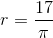### Example Question #9 : Circles

What is the diameter of a circle given the area of 30?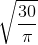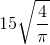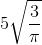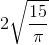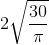Explanation:

Write the area formula of a circle.Substitute the area.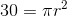Divide by pi on both sides.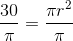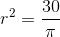Square root both sides.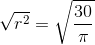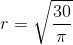The radius is:The diameter is double the radius.

The answer is:### Example Question #1 : Radius And Diameter

What is the diameter of a circle if the area is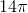?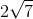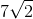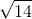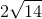Explanation:

Write the formula for the area of a circle.Substitute the area into the equation.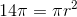Divide by pi on both sides.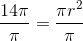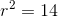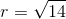The diameter is twice the radius.

The answer is:← Previous 1 3 4 5 6 7 8 9 17 18

### All GED Math Resources